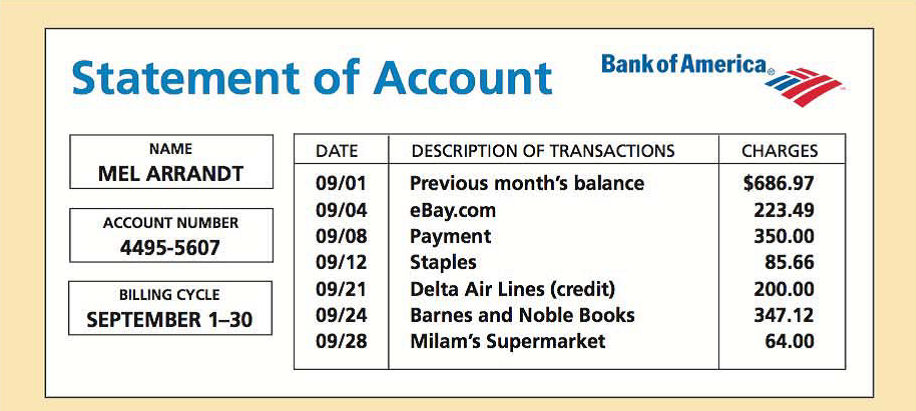Chapter 13, Problem 5AT### Contemporary Mathematics for Busin...

8th Edition
Robert Brechner + 1 other
ISBN: 9781305585447

#### Solutions

Chapter
Section### Contemporary Mathematics for Busin...

8th Edition
Robert Brechner + 1 other
ISBN: 9781305585447
Textbook Problem

# Mel Arrandt has a Bank of America account with a 13 % annual percentage rate calculated on the average daily balance. The billing date is the first day of each month, and the billing cycle is the number of days in that month.a. What is the average daily balance for September?b. What is the finance charge for September?c. What is Mel’s new balance?

(a)

To determine

To calculate: The average daily balance if Mel Arrandt has a Bank of America account with a 13% annual percentage rate and previous month balance is $686.97. The table showing the transactions is as follows:Explanation Given Information: Mel Arrandt has a Bank of America account with a 13% annual percentage rate and previous month balance is$686.97. The table showing the transactions is as follows:

Formula used:

The average daily balance is computed by dividing the total sum of daily balances by the number of days in a billing cycle.

Average daily balance=Sum of daily balancesdays in billing cycle

Calculation:

Previous month balance is $686.97. The table showing the sum of daily balances is as follows:  Dates No. of days Activity/ Amount Unpaid balance (amount+charge−payment) ($) Daily balances (unpaid balance×days) ($) September 1−3 3 Previous month balance$686.97 2,060.91 September 4−7 4 Charge 223.49 910.46 3,641.84 September 8−11 4 Payment 350 560

(b)

To determine

To calculate: The finance charge if Mel Arrandt has a Bank of America account with a 13% annual percentage rate and previous month balance is $686.97. Average daily balance is$694.76. The table showing the transactions is as follows:(c)

To determine

To calculate: The new balance if Mel Arrandt has a Bank of America account with a 13% annual percentage rate and previous month balance is $686.97. Average daily balance is$694.76, finance charge is $6.94, purchase and cash advance is$720.27 and payment and credit is \$550

The table showing the transactions is as follows:### Still sussing out bartleby?

Check out a sample textbook solution.

See a sample solution

#### The Solution to Your Study Problems

Bartleby provides explanations to thousands of textbook problems written by our experts, many with advanced degrees!

Get Started

#### Divide: 6x32x

Elementary Technical Mathematics

#### In Exercises 7-12, solve for y in terms of x. y24x2=2

Calculus: An Applied Approach (MindTap Course List)

#### Compute C7,7.

Understanding Basic Statistics

#### Define sampling with replacement and explain why is it used?

Statistics for The Behavioral Sciences (MindTap Course List)